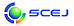\$B9V1i%W%m%0%i%`!J2q>l!&F|DxJL!K(B

English page

C \$B2q>l!&Bh(B 2 \$BF|(B

\$B:G=*99?7F|;~!'(B2015-03-05 09:42:37
\$B9V1i(B
\$B;~9o(B
\$B9V1i(B
\$BHV9f(B
\$B9V1iBjL\!?H/I=\$B%-!<%o!<%I(B\$BJ,N`(B
\$BHV9f(B
\$B\$BHV9f(B
\$BH?1~9)3X(B
(13:00\$B!A(B15:00) (\$B:BD9(B \$B:y0f(B \$B@?!&;3ED(B \$B??@!(B)
13:00\$B!A(B 13:20C213\$B4b<#NEMQ(BBNCT\$BAuCV%?!<%2%C%HIt\$N(Bin-situ\$B??6u>xCe(B/\$B%\$%*%sCmF~K!\$K\$h\$kCb2=\$K4X\$9\$k8&5f(B
(JAEA) (\$B@5(B)\$B@P;3(B \$B?7B@O:(B
BNCT
\$B%j%A%&%`(B
\$B\$,\$s<#NE(B
5-f86
13:20\$B!A(B 13:40C214\$BB?CJ3,%^%\$%/%m%j%"%/%?!<\$rMQ\$\$\$?B?5!G=%]%j%\$%_%IHyN3;R\$N:n@=(B
(\$B;:Am8&(B) (\$B@5(B)\$B!{@P:d(B \$B9'G7(B\$B!&(B \$B@P3@(B \$B8|(B\$B!&(B Chatterjee Maya\$B!&(B (\$B@5(B)\$BNkLZ(B \$BL@(B\$B!&(B \$BNkLZ(B \$BIR=E(B\$B!&(B (\$B@5(B)\$B@nGH(B \$BH%(B
polyimide
particles
multi-functionalization
5-f698
13:40\$B!A(B 14:00C215\$B%^%\$%/%m%j%"%/%?\$rMQ\$\$\$?%"%;%?!<%k\$*\$h\$S%[%k%^!<%k2=9gJ*\$N9g@.(B
(\$BF|L}(B) (\$B@5(B)\$B!{Cf;3(B \$B9@J?(B\$B!&(B (\$B@5(B)\$BB@ED(B \$B=SI'(B
Synthesis of Acetal and Formal compounds
microreactor
solid acid catalyst
5-f638
14:00\$B!A(B 14:20C216\$B%^%\$%/%m%j%"%/%?!<\$rMQ\$\$\$?%3%s%Q%/%H5\$1U8GH?1~%W%m%;%9\$N3+H/(B
(\$B5~Bg9)(B) (\$B3X(B)\$BK\B?(B \$BCN2B(B\$B!&(B (\$B@5(B)\$BJ!ED(B \$B5.;K(B\$B!&(B (\$B@5(B)\$B!{KR(B \$BBYJe(B\$B!&(B (\$B5~Bg4D2J%;(B) (\$B@5(B)\$BCf@n(B \$B9@9T(B\$B!&(B (\$B5~Bg9)(B) (\$B@5(B)\$BA0(B \$B0lW"(B
microreactor
packed-bed reactor
carbon catalyst
5-f556
14:20\$B!A(B 14:40C217\$B%^%\$%/%mN.O)Cf\$N%(%^%k%8%g%s\$NEE>l0u2C\$K\$h\$k2rF}2=\$K\$*\$h\$\\$9M-5!Aj\$N
(\$B:eI\Bg1!9)(B) (\$B@5(B)\$B!{IpF#(B \$BL@FA(B\$B!&(B (\$B3X(B)\$B>>K\(B \$BJ~G7(B\$B!&(B (\$B:eI\Bg9)(B) \$BJ?8}(B \$BM*0l(B\$B!&(B (\$B:eI\Bg1!9)(B) (\$B@5(B)\$BFAK\(B \$BM&?M(B
Demulsification
Electric field
Electrolyte
5-f378
14:40\$B!A(B 15:00C218\$B>K;@%\$%*%s\$N%^%\$%/%m4T85%7%9%F%`\$N3+H/(B
(\$BF|N)F|N)8&(B) (\$B@5(B)\$B!{@uLn(B \$BM32V;R(B\$B!&(B (\$BElBg9)(B) \$BM-N1(B \$B9nMN(B\$B!&(B \$B?{Ln(B \$B=\$(B\$B!&(B (\$BF|N)F|N)8&(B) (\$B@5(B)\$BIY3_(B \$B@9E5(B\$B!&(B (\$BF|N)%\$%s%U%i(B) \$B0KEl(B \$BA5(B\$B!&(B (\$BElBg9)(B) \$B;0Bp(B \$BN<(B
microreactor system for reduction
metal reductant
nitrate ion
5-f69
15:00\$B!A(B 15:20\$B5Y7F(B
(15:20\$B!A(B16:20) (\$B:BD9(B \$BKR(B \$BBYJe!&@uLn(B \$BM32V;R(B)
15:20\$B!A(B 15:40C220\$B%(%C%A%s%0%"%k%_%K%&%`:`NA\$rMQ\$\$\$?%^%\$%/%m%j%"%/%?\$N3+H/(B
(\$BG@9)Bg1!9)(B) (\$B3X(B)\$B!{J?>>(B \$B9(H~(B\$B!&(B (\$B3X(B)\$B>.;3(B \$BK'L\$(B\$B!&(B (\$B3X(B)\$B\$B!&(B (\$B@5(B)\$B:y0f(B \$B@?(B\$B!&(B (\$B@5(B)\$B55;3(B \$B=(M:(B
microreactor
structured catalyst
methanol reforming
5-f222
15:40\$B!A(B 16:00C221\$B1U1UCj=P\$K\$*\$1\$k%^%\$%/%mN.O)@_7WK!\$N3+H/(B
(\$B?@8M@=9]=j(B) (\$B@5(B)\$B!{H>C+(B \$B9-1{(B\$B!&(B (\$B@5(B)\$BF#_7(B \$B>4Mx(B\$B!&(B (\$B@5(B)\$B>>2,(B \$BN<(B\$B!&(B (\$B@5(B)\$BLn0l?'(B \$B8xFs(B
microchannel
extraction
mass transfer
5-f392
16:00\$B!A(B 16:20C222\$B30ItJBNs?(G^=
(\$B5~Bg1!9)(B) (\$B@5(B)\$B!{C+8}(B \$BCR(B\$B!&(B \$BB?2l(B \$BIRZv(B\$B!&(B (\$B@5(B)\$BEBB<(B \$B=\$(B\$B!&(B (\$B@5(B)\$BD9C+It(B \$B?-<#(B
parallelized microreactor
blockage detection
catalytic reaction
5-f406

\$B9V1iH/I=%W%m%0%i%`(B
\$B2=3X9)3X2q(B \$BBh(B80\$BG/2q(B(C) 2015 \$B8x1W
Most recent update: 2015-03-05 09:42:37
E-mail: inquiry-80awww3.scej.org
This page was generated byeasp 2.36; proghtml 2.37 (C)1999-2015 kawase